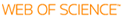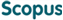### BROWSE

#### Related ResearcherJang, Bongsoo
Computational Mathematical Science Lab (CMS Lab)
Research Interests
• Numerical analysis, dynamics, fractional systems, data sciences

#### ITEM VIEW & DOWNLOAD

New explicit and accelerated techniques for solving fractional order differential equations

Cited 0 times inCited 0 times inTitle
New explicit and accelerated techniques for solving fractional order differential equations
Author
Issue Date
2020-08
Publisher
ELSEVIER SCIENCE INC
Citation
APPLIED MATHEMATICS AND COMPUTATION , v.379, pp.125228
Abstract
This paper proposes new direct and acceleration numerical methods for solving Fractional order Differential Equations (FDEs). For the Caputo differential operator with fractional order 0 < nu < 1, we rewrite the FDE as an equivalent integral form circumventing the derivative of the solution by the integral by parts. We obtain a discrete formulation directly from the integral form using the Lagrange interpolate polynomials. We provide truncation errors depending on the fractional order v for linear and quadratic interpolations, which are O(h(2-nu)) and O(h(3-nu)) respectively. In order to overcome a strong singular issue as nu approximate to 0 we propose the explicit predictor-corrector scheme with perturbation technique. In case of nu approximate to 1, the truncation errors are reduced by O(h) and O(h(2)). In order to accelerate the con- vergence rate, we decompose nu = nu/2 + nu/2, convert the FDE into the system of FDEs, and apply the predictor-corrector scheme. Numerical tests for linear, nonlinear, variable order and time-fractional sub-diffusion problems demonstrate that the proposed methods give a prominent performance. We also compare the numerical results with other high-order explicit and implicit schema. (C) 2020 Elsevier Inc. All rights reserved.
URI
https://scholarworks.unist.ac.kr/handle/201301/31977
URL
https://www.sciencedirect.com/science/article/pii/S0096300320301971
DOI
10.1016/j.amc.2020.125228
ISSN
0096-3003
Appears in Collections:
MTH_Journal Papers
Files in This Item:
There are no files associated with this item.

can give you direct access to the published full text of this article. (UNISTARs only)Items in DSpace are protected by copyright, with all rights reserved, unless otherwise indicated.